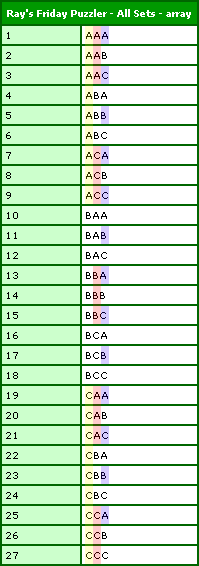# Ray's Friday Puzzler: Listing All Possibilities Of A Set

By on
Tags:

I finally got a chance to work on Ray's puzzler for April 27, 2007. It builds the result set by viewing the array as a table that has rows (set values) and columns (characters within a value). When you look at this way, you can build the array using a simple nested loop. The complicated part is using this RxC (row by column) index to figure out which character we are supposed to be using.

After analyzing the possible outcomes you will see that there are N^X possible values where N is the number of characters in the passed in set and X is the length of the each resultant item. This becomes our number of rows. Then, of course, the number of character columns is equal to the length of the set items (also passed id).

The alternating is a bit trickier to see. The last column has a new value for every row. The second to last column has a new value for every N rows (where N is the number of available characters). The third to last column has a new value for every N * N rows since it can only alternate after the second to last row has alternated.

You might begin to see the pattern is that a given column value alternates based on the length of the sets (X), the current column (C) and the number of characters (N) using this rule:

N^(X - C)

Once we know that, we can figure out which letter we are looking at based on the number of times our sequence has repeated and the current row. That equation is a bit complicated, so see the code below:

``````<cffunction
name="AllSets"
access="public"
returntype="array"
output="false"
hint="Given the list of characters and the desired length, an array of all possible value combinations will be returned.">

<!--- Define arguments. --->
<cfargument
name="Values"
type="string"
required="true"
hint="A comma delimited list of values."
/>

<cfargument
name="Length"
type="numeric"
required="true"
hint="The length of the resultant set values."
/>

<!--- Define the local scope. --->
<cfset var LOCAL = StructNew() />

<!---
Check to see if we have a valid set and a valid length.
We can only return resultant value if we have a value
set and length that is bigger than zero.
--->
<cfif (
(NOT Len( ARGUMENTS.Values )) OR
(ARGUMENTS.Length LTE 0)
)>

<!---
We have an invalid set or target length. To work
with this, just return an empty array.
--->
<cfreturn ArrayNew( 1 ) />

<!---
Check to see if the length is one. If it's just one,
then we don't have to do any work; just convert the
set to an array.
--->
<cfelseif (ARGUMENTS.Length EQ 1)>

<!--- Create and return an array from the list. --->
<cfreturn ListToArray(
ARGUMENTS.Values
) />

</cfif>

<!---
ASSERT: At this pointer, we know that we definitely
have a valid set of values and a valid target length
that is greater than one (this will actually require
value combinations).
--->

<!---
Convert the set to an array so that we can more eaily
access each letter.
--->
<cfset LOCAL.Values = ListToArray(
ARGUMENTS.Values
) />

<!--- Create an array to hold the set values. --->
<cfset LOCAL.Set = ArrayNew( 1 ) />

<!---
Loop over the rows to start populating each record.
We are going to be building one row at a time by
looping over each possible character. We know how
many records we have since each letter can be in
each spot.
--->
<cfloop
index="LOCAL.RowIndex"
from="1"
to="#(ListLen( ARGUMENTS.Values ) ^ ARGUMENTS.Length)#"
step="1">

<!---
Create a set value that will be added to the set
we are building. We are going to build the value
out of the set first to make the code more readable.
--->
<cfset LOCAL.Value = "" />

<!---
Loop over each character in the string. We know
how many characters we need based on the length
that was passed in.
--->
<cfloop
index="LOCAL.ColumnIndex"
from="1"
to="#ARGUMENTS.Length#"
step="1">

<!---
Get the MOD Toggle index for this column. Each
column value toggles (switches to the next
character) based on a MOD index.
--->
<cfset LOCAL.ModIndex = (
ArrayLen( LOCAL.Values ) ^
(ARGUMENTS.Length - LOCAL.ColumnIndex)
) />

<!---
Get the bucket that we are in. By bucket, I mean
which "iteration" are we in, in which this value
is different form the previous letter.
--->
<cfset LOCAL.Bucket = Ceiling(
LOCAL.RowIndex / LOCAL.ModIndex
) />

<!---
Once we have the bucket, we can use that and the
number of available values to figure out which
letter we should be pointing to.
--->
<cfset LOCAL.ValueIndex = (((LOCAL.Bucket - 1) MOD ArrayLen( LOCAL.Values )) + 1) />

<!---
Get the new value character out of our working
set based on the value index we just calculated.
--->
<cfset LOCAL.Value = (
LOCAL.Value &
LOCAL.Values[ LOCAL.ValueIndex ]
) />

</cfloop>

<!--- Append the value to the results array. --->
<cfset ArrayAppend(
LOCAL.Set,
LOCAL.Value
) />

</cfloop>

<!--- Return the results array. --->
<cfreturn LOCAL.Set />
</cffunction>
``````

This uses two loops and no recursion, but is certainly a bit verbose. And now, to test; running this:

``````<cfset arrSet = AllSets(
"A,B,C",
3
) />

<cfdump
var="#arrSet#"
label="Ray's Friday Puzzler - All Sets"
/>
``````

... we get the following CFDump output:I have color coded the alternating rows to help see where things are changing.

Want to use code from this post? Check out the license.Nice solution. I was working on something very similar by using the exponent to determine total possibilities and two loops, but I got a little stuck towards the end... I'm glad you solved it this way so that I can see where I went wrong.

Dan@Dan,

Thanks. Yeah, that MOD equation was driving me CRAZY for a while. I kept getting the sequence "1,2,0,1,2,0" and I wanted "1,2,3,1,2,3"!!!!